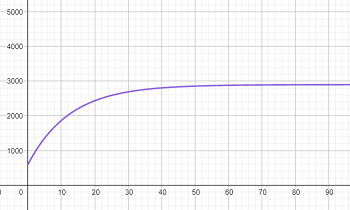# Universal instruments found that the monthly demand for its new line of Galaxy Home Computers t...

## Question:

Universal instruments found that the monthly demand for its new line of Galaxy Home Computers t months after placing the line on the market was given by {eq}D(t) = 2900 - 2300e^{-0.08t} (t > 0). {/eq} Graph this function and answer the following questions and find what is the demand after 1 month. After 1 year? After 2 years? after 5 years?

## Graphs:

At the end of the day, a demand function is just another function, and can be treated like any other function having its properties. One of the most powerful tools we have for understanding what a function is really telling us is to graph it. We will graph the given demand function, then use the graph to answer the questions.

Our demand function is plotted below.{eq}\begin{align*} D(t) = 2900 - 2300e^{-0.08t} \end{align*} {/eq}

We use the trace function to find that after 1 month, the demand is (rounded to the nearest whole number)

{eq}\begin{align*} D(1) &\approx 777 \end{align*} {/eq}

Then 1 year is 12 months, so after 1 year the demand is

{eq}\begin{align*} D(12) &\approx 2,019 \end{align*} {/eq}

2 years is 24 months, so after 2 years the demand is

{eq}\begin{align*} D(24) &\approx 2, 563 \end{align*} {/eq}

Lastly, 5 years is 60 months, so after 5 years the demand is

{eq}\begin{align*} D(60) &\approx 2, 881 \end{align*} {/eq}